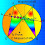## Saturday, September 7, 2013

### Geometry Problem 919: Rhombus, Triangle, Perpendicular, 90 Degrees, Congruence

Geometry Problem
Post your solution in the comment box below

Level: Mathematics Education, High School, Honors Geometry, College.

Click the figure below to see the complete problem 919.1.Let a denote the length of each side of the rhombus.
BD bisects angle ABC
Let h be the distance of D from BA or BC.
Clearly MG = h = HP.
(i.e.)FG - FM = EP - EH
So FG + EH = EP + FM

2.Rewrite the statement to FG-FM = EP-EN
Both sides equals to the height of the rhombus, thus proved.

3.FM + EN = FM + EN, GM = PN from being altitude of rombus
(GM + MF) + EN = FM + (PN + NE)
GF + EN = EP + FM

To Antonio: I think the problem I suggested a few days ago is more interesting that these problems. However, it is your right to make the selection.
Erina-NJ

1.